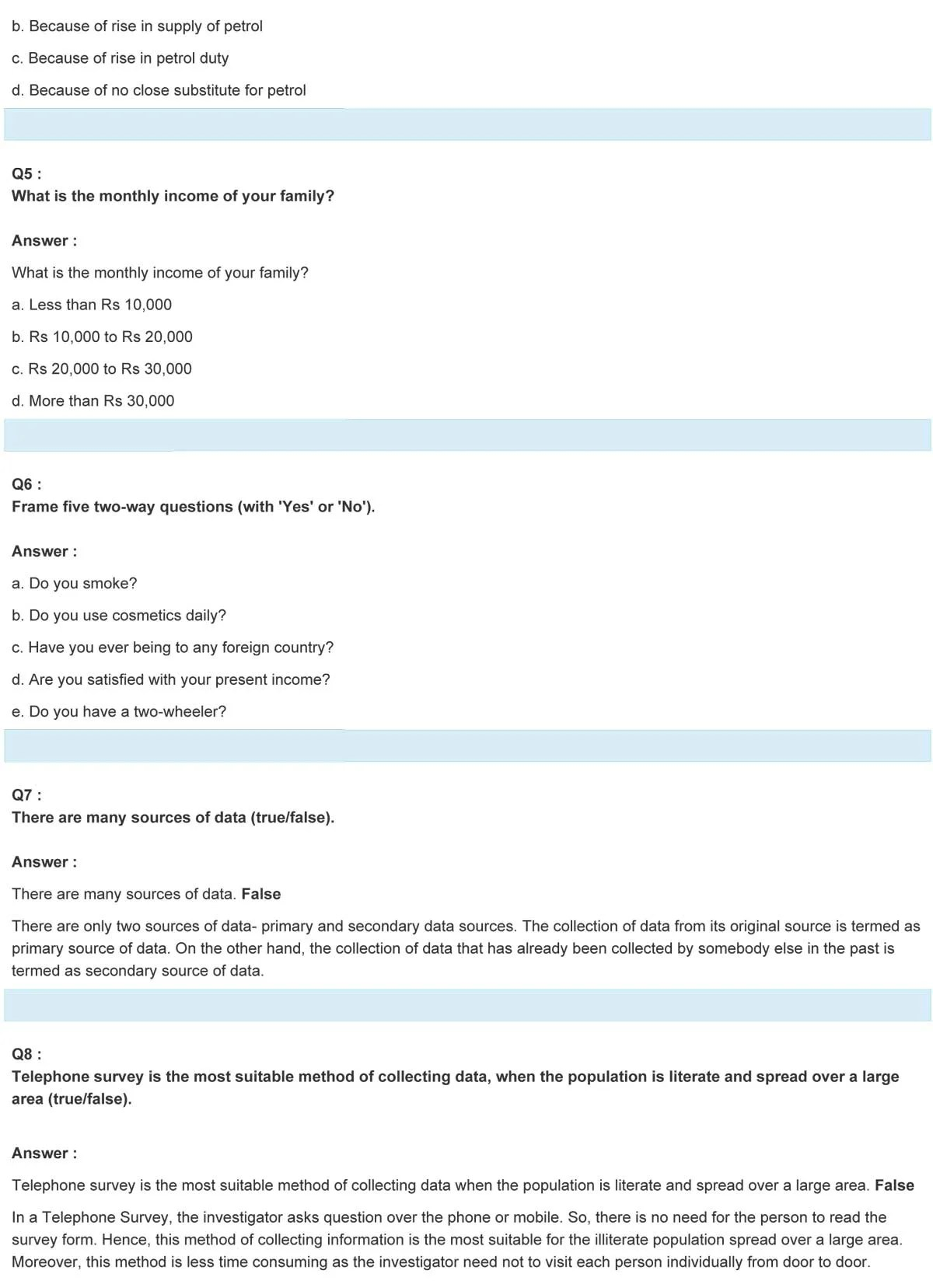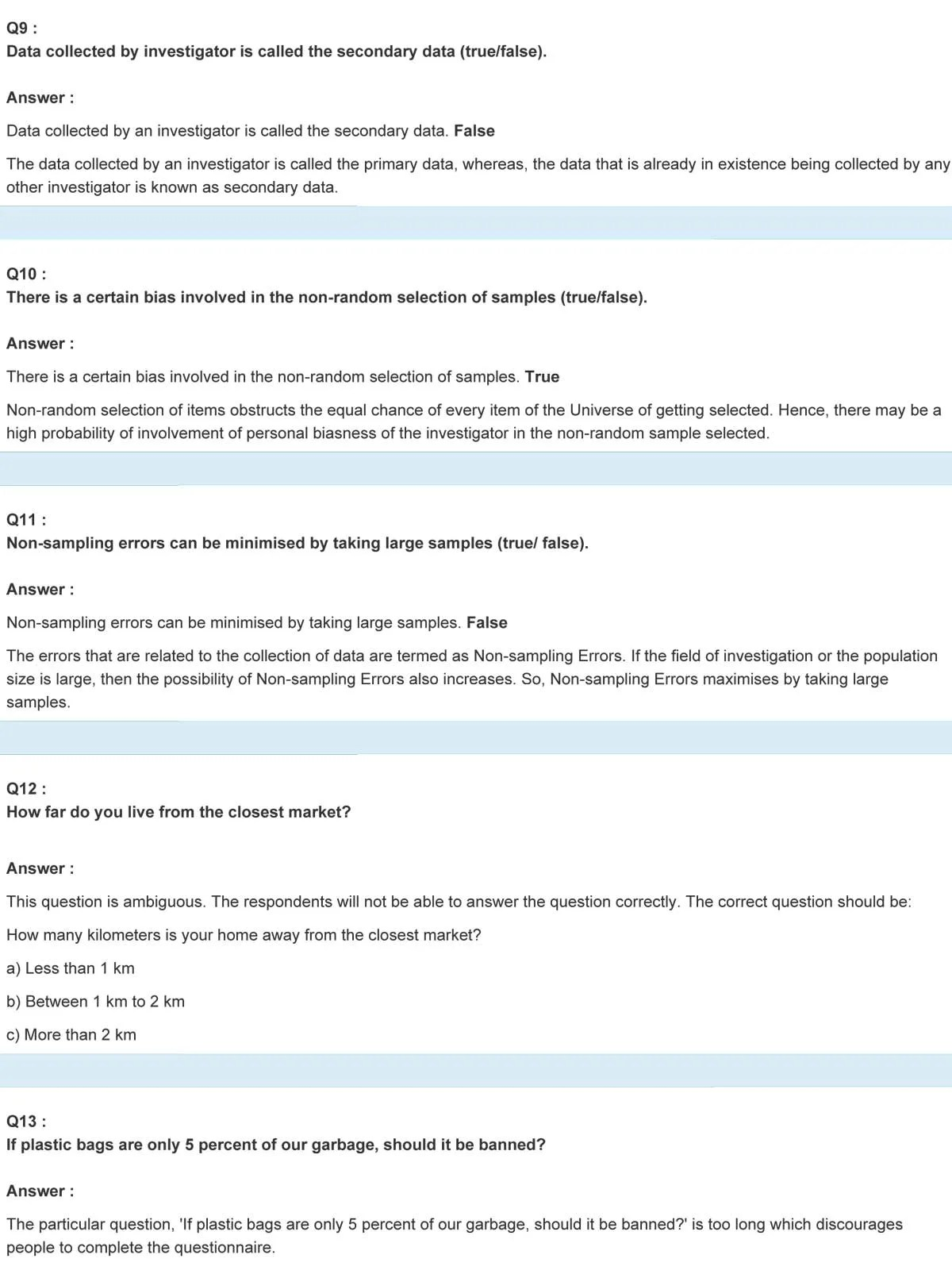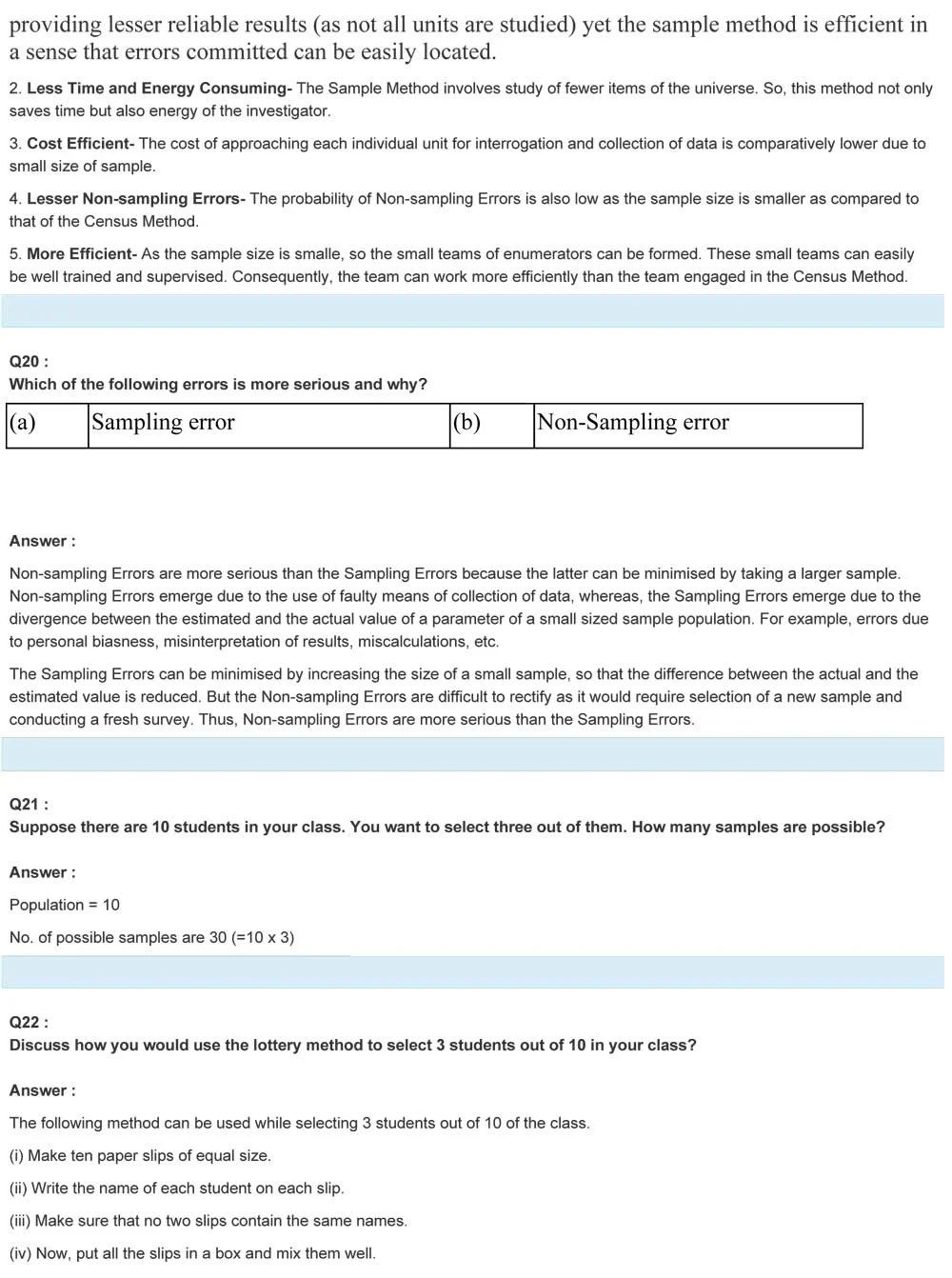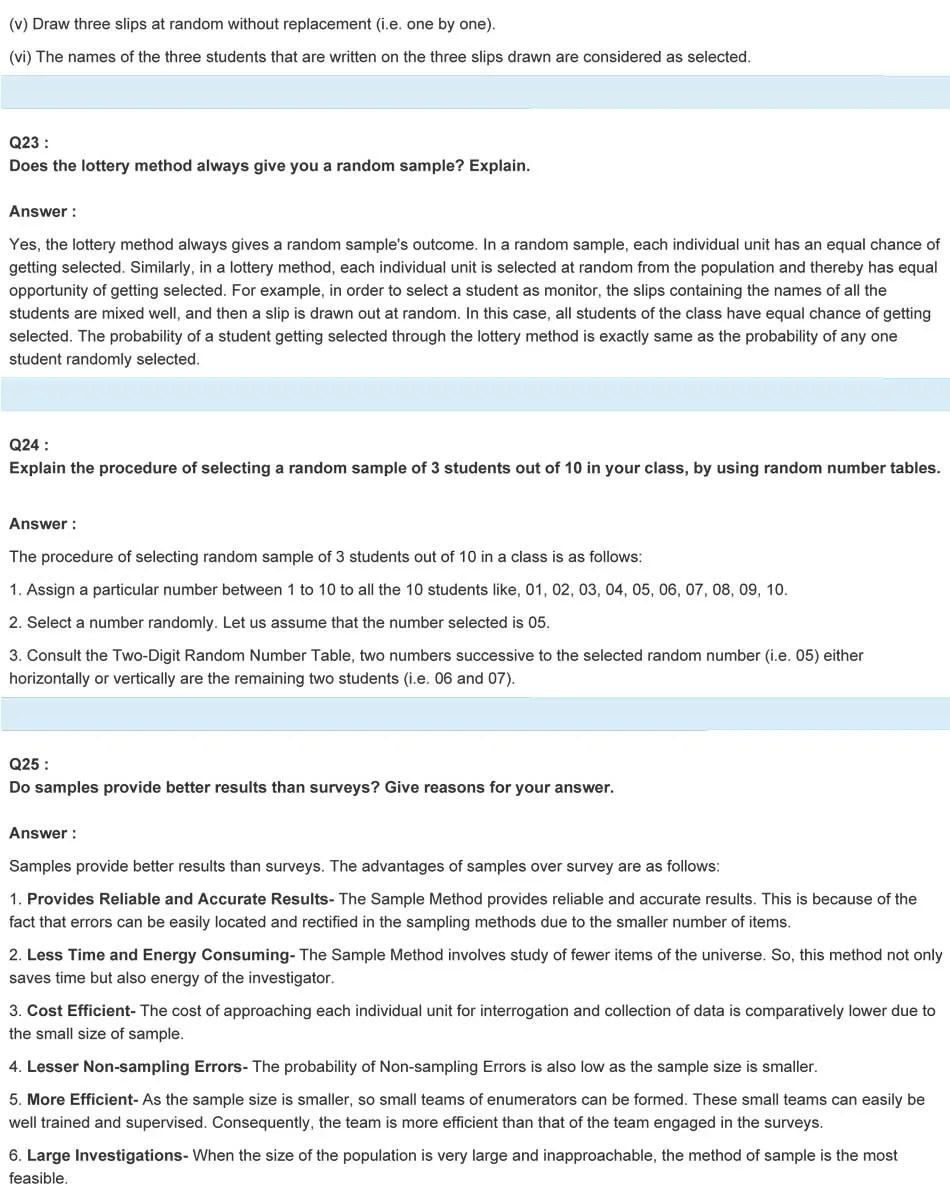# NCERT Solutions For Class 11 Chapter 2 Statistics

### NCERT Solutions Class 11 Statistics Chapter 2

NCERT Solutions are known to be a helpful book while studying for the CBSE Class 11 Statistics examinations. This study material possesses intense knowledge and the solutions collected by the subject matter experts are beneficial for the students.

NCERT Solutions Class 11 Statistics Chapter 2 Collection Of Data provides us with useful data to all the topics. These NCERT books assist the students to score well in their examinations. The students have to learn the basic fundamentals of the subject Statistics in class 11; NCERT books explain all the concepts in a comprehensive way.

## Concepts covered in this chapter –

• Introduction
• What are the sources of data?
• How do we collect the data?
• Preparation of Instrument
• Mode of Data Collection
• Pilot Survey

Class 11 NCERT Solutions for Statistics chapter 2 provides a wide degree of illustrative examples; which helps the students to understand and learn quickly. For more solutions and study materials Statistics , refer NCERT solutions class 11 Statistics.

#### NCERT Solutions for Class 11 Economics Statistics

for Economics Chapter 2

Collection of Data

Exercise: Solutions of Questions on Page Number: 20

Q1: Which of the following is the most important when you buy a new dress?

Answer: Which of the following is the most important when you buy a new dress?

a. Colour

b. Brand

c. Price

d. Size

Q2: How often do you use computers?

Answer: How often do you use computers?

a. Less than 1 hour a day

b. 1 to 3 hours a day

c. 3 to 5 hours a day

d. More than 5 hours

Q3: Which of the following newspaper/s do you read regularly?

a. The Times of India

b. The Hindustan Times

c. Indian Express

d. The Tribune

Q4: Rise in the price of petrol is justified.

Answer: Rise in the price of petrol is justified.

a. Because of rising in demand for petrol

b. Because of rising in the supply of petrol

c. Because of rising in petrol duty d. Because of no close substitute for petrol

Q5. What is the monthly income of your family?

a. Less than Rs 10,000

b. Rs 10,000 to Rs 20,000

c. Rs 20,000 to Rs 30,000

d. More than Rs 30,000

Q6: Frame five two-way questions (with ‘Yes’ or ‘No’).

a. Do you smoke?

b. Do you use cosmetics daily?

c. Have you ever being to any foreign country?

d. Are you satisfied with your present income?

e. Do you have a two-wheeler?

Q7: There are many sources of data (true/false).

There are many sources of data. False
There are only two sources of data- primary and secondary data sources. The collection of data from its original source is termed as the primary source of data. On the other hand, the collection of data that has already been collected by somebody else in the past is termed as a secondary source of data.

Q8: Telephone survey is the most suitable method of collecting data when the population is literate and spread over a large area (true/false).

The telephone survey is the most suitable method of collecting data when the population is literate and spread over a large area. False

In a Telephone Survey, the investigator asks the question over the phone or mobile. So, there is no need for the person to read the survey form. Hence, this method of collecting information is the most suitable for the illiterate population spread over a large area. Moreover, this method is less time consuming as the investigator need not visit each person individually from door to door.

Q9: Data collected by the investigator is called the secondary data (true/false).

Data collected by an investigator is called the secondary data. False
The data collected by an investigator is called the primary data, whereas, the data that is already in existence being collected by any other investigator is known as secondary data.

Q10: There is a certain bias involved in the non-random selection of samples (true/false).
There is a certain bias involved in the non-random selection of samples. True

Non-random selection of items obstructs the equal chance of every item of the Universe of getting selected. Hence, there may be a high probability of involvement of personal biases of the investigator in the non-random sample selected.

Q11: Non-sampling errors can be minimised by taking large samples (true/ false).

Non-sampling errors can be minimised by taking large samples. False

The errors that are related to the collection of data are termed as Non-sampling Errors. If the field of investigation or the population size is large, then the possibility of Non-sampling Errors also increases. So, Non-sampling Errors maximises by taking large samples.

Q12: How far do you live from the closest market?

Answer: This question is ambiguous. The respondents will not be able to answer the question correctly. The correct question should be:

How many kilometres is your home away from the closest market?

a) Less than 1 km

b) Between 1 km to 2 km

c) More than 2 km

Q13: If plastic bags are only 5 per cent of our garbage, should it be banned?

The particular question, ‘If plastic bags are only 5 per cent of our garbage, should it be banned?’ is too long which discourages people to complete the questionnaire.

The correct question should be:

Do you think the use of plastic bags should be banned?

a) Yes

b) No

Q14: Wouldn’t you be opposed to increasing in price of petrol?

Although the answer to this question needs a wider view and knowledge of the economic condition and far-reaching effect of rising in the petrol price, the majority of people will argue against such a rise. Thus, the more effective question could be:

Do you think the hike in prices of petrol is justified?

a) Yes

b) No

Q15 :

(a) Do you agree with the use of chemical fertilisers?

(b) Do you use fertilisers in your fields?

(c) What is the yield per hectare in your field?

The chronological order of the questions asked is incorrect. The order lacks the direction of causation. It should move from general to specific that makes the respondents easy and comfortable

(i) The correct order of the above questions should be: What is the yield per hectare in your field?

(ii) Do you use fertilisers in your fields?

(iii) Do you agree with the use of chemical fertilisers?

Q16: You want to research the popularity of Vegetable Atta Noodles among children. Design a suitable questionnaire for collecting this information.

Q17: In a village of 200 farms, a study was conducted to find the cropping pattern. Out of the 50 farms surveyed, 50% grew only wheat. Identify the population and the sample here.

Answer: Population refers to the aggregate or the total items to be studied for an investigation. So, the population here is 200 farms. The sample is a subset of the population. In other words, a small set selected from the population for the statistical study is referred to as a sample population. Out of 200 farms, only 50 farms are selected for a survey; therefore, the sample population is 50 farms.

Q18: Give two examples each of sample, population and variable.

The sample is a subset of the population. In other words, a small set selected from the population for the statistical study is referred to as the sample population. For example, in order to study the growth pattern of students, the heights of 50 students (sample) are recorded from a school of 500 students (population). Similarly, in order to record the level of sugar in the blood, the blood sample of 2000 people (sample) was taken from 20,000 people (population).
Population refers to the aggregate or the total items to be studied for an investigation. In the above examples, 500 students and 20,000 people constitute the population.
Variables are the characteristics of a sample or population that can be expressed in numbers such as, height, income, age, etc.

019: Which of the following methods give better results and why?
(a) Census (b) Sample

Sample Method gives better results than the Census Method due to the following reasons.

1. Accuracy- Although census method provides more accurate and reliable results as compared to the sampling method but in the sampling method, the errors can be easily located and rectified in the sampling methods due to the smaller number of items. Therefore, despite the sampling method providing lesser reliable results (as not all units are studied) yet the sampling method is efficient in a sense that errors committed can be easily located.

2. Less Time and Energy Consuming- The Sample Method involves the study of fewer items of the universe. So, this method not only saves time but also the energy of the investigator.

3. Cost Efficient- The cost of approaching each individual unit for interrogation and collection of data is comparatively lower due to the small size of a sample.

4. Lesser Non-sampling Errors- The probability of Non-sampling Errors is also low as the sample size is smaller as compared to that of the Census Method.

5. More Efficient- As the sample size is smaller, so the small teams of enumerators can be formed. These small teams can easily be well trained and supervised. Consequently, the team can work more efficiently than the team engaged in the Census Method.

Q20: Which of the following errors is more serious and why?

 (a) Sampling error (b) Non-Sampling error

Non-sampling errors are more serious than the Sampling Errors because the latter can be minimised by taking a larger sample. Non-sampling Errors emerge due to the use of faulty means of a collection of data, whereas, the Sampling Errors emerge due to the divergence between the estimated and the actual value of a parameter of a small-sized sample population. For example, errors due to personal biases, misinterpretation of results, miscalculations, etc. The Sampling Errors can be minimised by increasing the size of a small sample so that the difference between the actual and the estimated value is reduced. But the Non-sampling Errors are difficult to rectify as it would require selection of a new sample and conducting a fresh survey. Thus, Non-sampling Errors are more serious than Sampling Errors.

Q21: Suppose there are 10 students in your class. You want to select three out of them. How many samples are possible?

Answer : Population = 10 No. of possible samples are 30 (=10 x 3)

Q22: Discuss how you would use the lottery method to select 3 students out of 10 in your class?
Answer: The following method can be used while selecting 3 students out of 10 of the class.

(i) Make ten paper slips of equal size.

(ii) Write the name of each student on each slip.

(iii) Make sure that no two slips contain the same names.

(iv) Now, put all the slips in a box and mix them well.

(v) Draw three slips at random without replacement (i.e. one by one).

(vi) The names of the three students that are written on the three slips drawn are considered as selected.

Q23: Does the lottery method always give you a random sample? Explain.

Yes, the lottery method always gives a random sample’s outcome. In a random sample, each individual unit has an equal chance of getting selected. Similarly, in a lottery method, each individual unit is selected at random from the population and thereby has an equal opportunity of getting selected. For example, in order to select a student as a monitor, the slips containing the names of all the students are mixed well, and then a slip is drawn out at random. In this case, all students in the class have an equal chance of getting selected. The probability of a student getting selected through the lottery method is exactly the same as the probability of any one student randomly selected.

Q24: Explain the procedure of selecting a random sample of 3 students out of 10 in your class, by using random number tables.

The procedure for selecting a random sample of 3 students out of 10 in a class is as follows:

1. Assign a particular number between 1 to 10 to all the 10 students Ike, 01, 02, 03, 04, 05, 06, 07, 08, 09, 10.

2. Select a number randomly. Let us assume that the number selected is 05.

3. Consult the Two-Digit Random Number Table, two numbers successive to the selected random number (i.e. 05) either horizontally or vertically are the remaining two students (i.e. 06 and 07).

Q25: Do samples provide better results than surveys? Give reasons for your answer.

Samples provide better results than surveys. The advantages of samples over the survey are as follows:

1. Provides Reliable and Accurate Results- The Sample Method provides reliable and accurate results. This is because of the fact that errors can be easily located and rectified in the sampling methods due to the smaller number of items.

2. Less Time and Energy Consuming- The Sample Method involves the study of fewer items of the universe. So, this method not only saves time but also the energy of the investigator.

3. Cost Efficient- The cost of approaching each individual unit for interrogation and collection of data is comparatively lower due to the small size of the sample.

4. Lesser Non-sampling Errors- The probability of Non-sampling Errors is also low as the sample size is smaller.

5. More Efficient- As the sample size is smaller, so small teams of enumerators can be formed. These small teams can easily be well trained and supervised. Consequently, the team is more efficient than that of the tease engaged in the surveys.

6. Large Investigations- When the size of the population is very large and inapproachable, the method of sample is the most feasible.